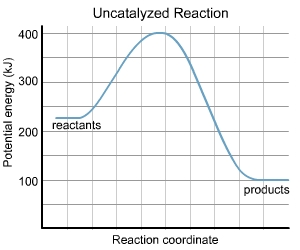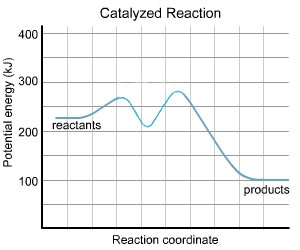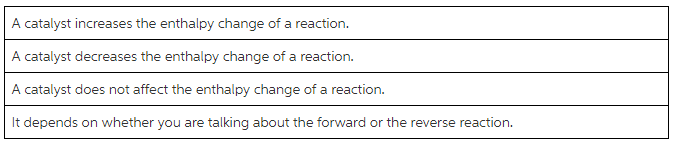# What is the value of the activation energy of the uncatalyzed reaction?Part A

What is the value of the activation energy of the uncatalyzed reaction?
Express your answer to three significant figures and include the appropriate units.

Part B

What is the value of the enthalpy change of the uncatalyzed reaction?
Express your answer to three significant figures and include the appropriate units.

Part C
What is the value of the activation energy of the uncatalyzed reaction in reverse?

Part D

What is the value of the enthalpy change of the uncatalyzed reaction in reverse?
Express your answer to three significant figures and include the appropriate units.

Part E

How does the presence of a catalyst affect the activation energy of a reaction?
How does the presence of a catalyst affect the activation energy of a reaction?Part F

How does the presence of a catalyst affect the enthalpy change of a reaction?
How does the presence of a catalyst affect the enthalpy change of a reaction?For the uncatalysed reaction

The threshold energy = 400kJ

E reactants = 250kJ

E products = 100kJ

Thus activation energy Ea = Ethreshold - E reactans

and enthalpy of reaction Delta H = E products - E reactants

Part A

Activation energy for uncatalysed reaaction = E thre shold - E rreactant

= 400 -250 = 150kJ

Part B

enthalpy of reaction = 100-250

= - 150kJ

exothermic reaction

Part C for the reverse reaction

activation eenrgy = 400-100

= 300kJ

Part D

delta H for reverse reaction = 250 -100

= + 150 kJ

Part E

A catalyst lowers the activation energy of the reaction by altering the reaction pathway(mechanism)

Part F

The presence of catalyst does not change the enthalpy of reaction. It depnds on the energies of reactants andproducts which are unaffected by a catalst.